# tree

## A gold-grabbing game ★★

Author(s): Rosenfeld

Setup Fix a treeand for every vertex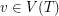a non-negative integer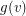which we think of as the amount of gold at.

2-Player game Players alternate turns. On each turn, a player chooses a leaf vertexof the tree, takes the gold at this vertex, and then deletes. The game ends when the tree is empty, and the winner is the player who has accumulated the most gold.

Problem   Find optimal strategies for the players.

Keywords: game; tree

## Graphs with a forbidden induced tree are chi-bounded ★★★

Author(s): Gyarfas

Say that a family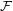of graphs is-bounded if there exists a functionso that every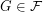satisfies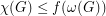.

Conjecture   For every fixed tree, the family of graphs with no induced subgraph isomorphic tois-bounded.

Keywords: chi-bounded; coloring; excluded subgraph; tree

## Does the chromatic symmetric function distinguish between trees? ★★

Author(s): Stanley

Problem   Do there exist non-isomorphic trees which have the same chromatic symmetric function?

Keywords: chromatic polynomial; symmetric function; tree

## Graham's conjecture on tree reconstruction ★★

Author(s): Graham

Problem   for every graph, we letdenote the line graph of. Given thatis a tree, can we determine it from the integer sequence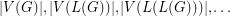?

Keywords: reconstruction; tree